## How to Convert Square Miles to Square Millimeters | Area Units

The image below represents square miles to square millimeters.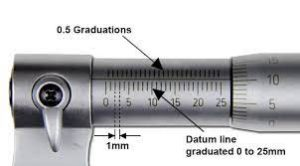To convert square miles to square millimeters, one essential parameter is needed and this parameter is Unit Value.

The formula for converting square miles to square millimeters:

Let’s solve an example;
Find the conversion of square miles to square millimeters when the unit value is 6.

1 Square Mile = 2589988110336 Square Millimeters

Then,

6 Square Miles = (6 x 2589988110336) Square Millimeters
6 Square Miles = 15539928662016 Square Millimeters

Therefore, the square millimeter(s) is 15539928662016.

## How to Convert Acres to Square Centimeters | Area Units

The image below represents acres to square centimeters.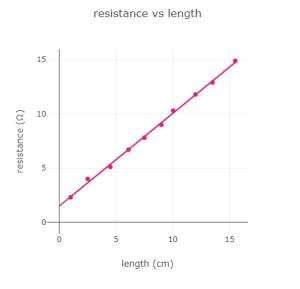To convert acres to square centimeters, one essential parameter is needed and this parameter is Unit Value.

The formula for converting acres to square centimeters:

1 Acre = 40468564 Square Centimeters

Let’s solve an example;
Find the conversion of acres to square centimeters when the unit value is 4.

1 Acre = 40468564 Square Centimeters

Then,

4 Acres = (4 x 40468564) Square Centimeters
4 Acres = 161874256 Square Centimeters

Therefore, the square centimeter(s) is 161874256.

## How to Convert Acres to Square Millimeters | Area Units

The image below represents acres to square millimeters.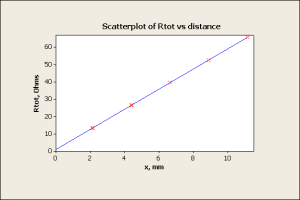To convert acres to square millimeters, one essential parameter is needed and this parameter is Unit Value.

The formula for converting acres to square millimeters:

Let’s solve an example;
Find the conversion of acres to square millimeters when the unit value is 3.

1 Acre = 4046856422 Square Millimeters

Then,

3 Acres = (3 x 4046856422) Square Millimeters
3 Acres = 12140569266 Square Millimeters

Therefore, the square millimeter(s) is 12140569266.

## How to Convert Square Yards to Square Millimeters | Area Units

The image below represents square yards to square millimeters.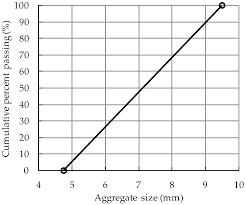To convert square yards to square millimeters, one essential parameter is needed and this parameter is Unit Value.

The formula for converting square yards to square millimeters:

1 Square Yard = 836127.4 Square Millimeters

Let’s solve an example;
Find the conversion of square yards to square millimeters when the unit value is 8.

1 Square Yard = 836127.4 Square Millimeters

Then,

8 Square Yards = (8 x 836127.4) Square Millimeters
8 Square Yards = 6689019.2 Square Millimeters

Therefore, the square millimeter(s) is 6689019.2.

## How to Convert Square Feet to Square Millimeters | Area Units

The image below represents square feet to square millimeters.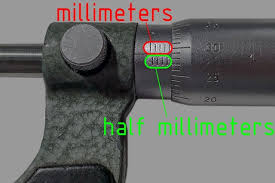To convert square feet to square millimeters, one essential parameter is needed and this parameter is Unit Value.

The formula for converting square feet to square millimeters:

1 Square Feet = 92903.04 Square Millimeters

Given an example;
Find the conversion of square feet to square millimeters when the unit value is 16.

1 Square Feet = 92903.04 Square Millimeters

Then,

16 Square Feet = (16 x 92903.04) Square Millimeters
16 Square Feet = 1486448.64 Square Millimeters

Therefore, the square millimeter(s) is 1486448.64.

## How to Convert Square Inches to Square Millimeters | Area Units

The image below represents square inches to square millimeters.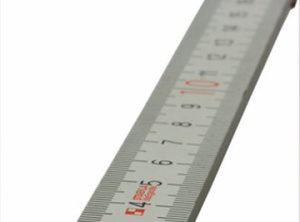To convert square inches to square millimeters, one essential parameter is needed and this parameter is Unit Value.

The formula for converting square inches to square millimeters:

1 Square Inch = 645.16 Square Millimeters

Given an example;
Convert square inches to square millimeters when the unit value is 8.

1 Square Inch = 645.16 Square Millimeters

Then,

8 Square Inches = (8 x 645.16) Square Millimeters
8 Square Inches = 5161.28 Square Millimeters

Therefore, the square millimeter(s) is 5161.28

## How to Convert Square Kilometers to Square Millimeters | Area Units

The image below represents square kilometers to square millimeters.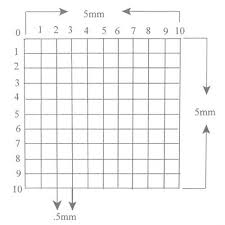The conversion of square kilometers to square millimeters requires one essential parameter and this parameter is Unit Value.

The formula for converting square kilometers to Square millimeters:

1 Square Kilometer = 1000000000000 Square Millimeters

Let’s solve an example;
Find the conversion of square kilometers to square millimeters when the unit value is 13.

1 Square Kilometer = 1000000000000 Square Millimeters

Then,

13 Square Kilometers = (13 x 1000000000000) Square Millimeters
13 Square Kilometers = 13000000000000 Square Millimeters

Therefore, the square millimeter(s) is 13000000000000.

## How to Convert Hectares to Square Millimeters | Area Units

The image below represents hectares to square millimeters.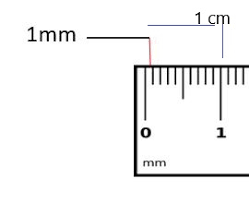The conversion of hectares to square millimeters requires one essential parameter and this parameter is Unit Value.

The formula for converting hectares to square millimeters:

1 Hectare = 10000000000 Square Millimeters

Given an example;
Convert hectares to square millimeters when the unit value is 12.

1 Hectare = 10000000000 Square Millimeters

Then,

12 Hectares = (12 x 10000000000) Square Millimeters
12 Hectares = 120000000000 Square Millimeters

Therefore, the square millimeter(s) is 120000000000.

## How to Convert Square Meters to Square Millimeters | Area Units

The image below represents square meters to square millimeters.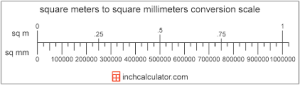The conversion of square meters to square millimeters requires one essential parameter and this parameter is Unit Value.

The formula for converting square meters to square millimeters:

1 Square Meter = 1000000 Square Millimeters

Let’s solve an example;
Convert square meters to square millimeters when the unit value is 4.

1 Square Meter = 1000000 Square Millimeters

Then,

4 Square Meters = (4 x 1000000) Square Millimeters
4 Square Meters = 4000000 Square Millimeters

Therefore, the square millimeter(s) is 4000000.

## How to Convert Square Centimeters to Square Millimeter | Area Units

The image below represents square centimeters to square millimeters.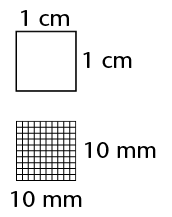The conversion of square centimeters to square millimeters requires one essential parameter and this parameter is Unit Value.

The formula for converting square centimeters to square millimeters:

1 Square Centimeter = 100 Square Millimeters

Let’s solve an example;
Find the conversion of square centimeters to square millimeters when the unit value is 4.

1 Square Centimeter = 100 Square Millimeters

Then,

4 Square Centimeters = (4 x 100) Square Millimeters
4 Square Centimeters = 400 Square Millimeters

Therefore, the square millimeter(s) is 400.